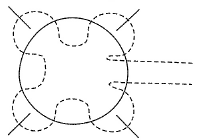Courses

# Test: Bohr's Theory Of H - Atom

## 19 Questions MCQ Test Chemistry for JEE | Test: Bohr's Theory Of H - Atom

Description
This mock test of Test: Bohr's Theory Of H - Atom for JEE helps you for every JEE entrance exam. This contains 19 Multiple Choice Questions for JEE Test: Bohr's Theory Of H - Atom (mcq) to study with solutions a complete question bank. The solved questions answers in this Test: Bohr's Theory Of H - Atom quiz give you a good mix of easy questions and tough questions. JEE students definitely take this Test: Bohr's Theory Of H - Atom exercise for a better result in the exam. You can find other Test: Bohr's Theory Of H - Atom extra questions, long questions & short questions for JEE on EduRev as well by searching above.
QUESTION: 1

### Which of the following is correct?

Solution:

Because isotones means the same number of neutron, So, from the question, option c is right, number of neutron in k is,39-19= 20,and,the number of neutron in ca is also 40-20=20 so it is isotones.

QUESTION: 2

### ‘Hartree’ is the atomic unit of energy and is equal to

Solution:

The potential energy of an electron in the first Bohr’s orbit in the H-atom is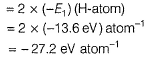This energy is defined as an atomic unit of energy called HARTREE.

QUESTION: 3

### Wave number of a spectral line for a given transition is x cm-1 for He+, then its value for Be3+ (isoelectronic of He+)for the same transition is

Solution: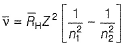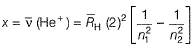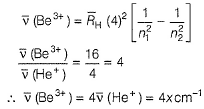QUESTION: 4

Which of the following electronic transitions requires that the greatest quantity of energy be absorbed by a hydrogen atom ?

Solution: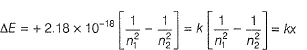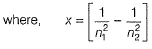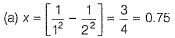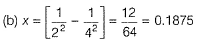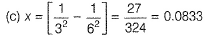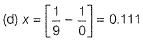Therefore, electronic transition (a) requires greatest quantity of energy.

QUESTION: 5

An electron in H-atom in its ground state absorbs 1.5 times as much as energy as the minimum required for its escape from the atom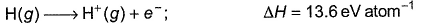Q.

Thus, kinetic energy given to the emitted electron is

Solution:

E1 = Energy of H-atom in the ground state = 13.6 eV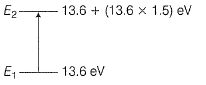Energy absorbed = (13.6 x 1.5) = 20.4 eV
E2 = Energy of the excited state
= 13.6+ 20.4= 34.0 eV
ΔE = KE = (E2 - E1)
= 34.0 - 13.6 = 20.4 eV

QUESTION: 6

Ionisation energy of He+ is 19.6x10-18 J atom -1. The energy of the first stationary state (n = 1)of Li2+ is

Solution:

Ionisation energy = - Energy of the electron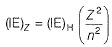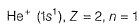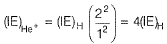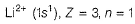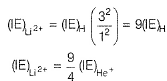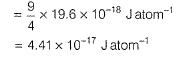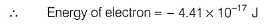QUESTION: 7

The kinetic energy of an electron in the second Bohr orbit of a hydrogen atom is (a0 is Bohr radius)

[AIEEE 2012]

Solution: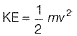Also, angular momentum is quantised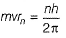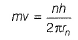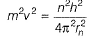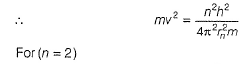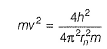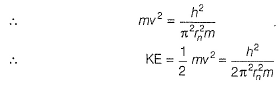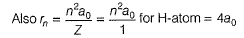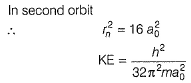QUESTION: 8

Energy of the electron in nth orbit is given by E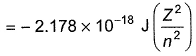Wavelength of light required to excite an electron in an H-atom from level n = 1 to n = 2 will be (h = 6.62 x 10-34 J s ; c = 3.0 x 108ms -1)

[AIEEE 2012]

Solution:

For H - atom , Z = 1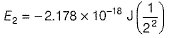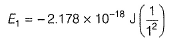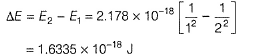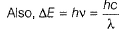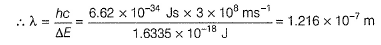QUESTION: 9

The potential energy of an electron in the second Bohr's orbit of the he±

Solution:

QUESTION: 10

In Lyman series, shortest wavelength of H-atom appears at x m, then longest wavelength in Balmer series of He+ appear at

Solution:

For the spectral line in H -atom and H -like species (one electron)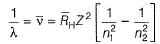For Lyman series
For shortest wavelength (maximum wave number) n2 → ∞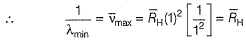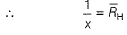For longest wave length (minimum wave number), n2 = (n1, + 1).
For Balmer series, n1 = 2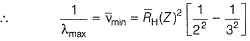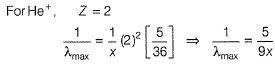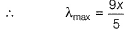QUESTION: 11

If the radius of the first Bohr orbit is x, then de-Broglie wavelength of the electron in the third orbit is nearly

Solution:

Angular momentum is quantised , hence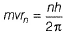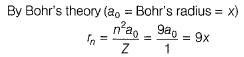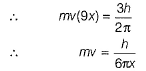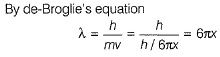QUESTION: 12

Direction (Q. Nos. 12-13) This section contains a paragraph, wach describing  theory, experiments, data etc. three Questions related to paragraph have been given.Each question have only one correct answer among the four given  ptions  (a),(b),(c),(d)

Radius of Bohr’s orbit of H-atom is 52.9 pm. An emission in H-atom starts from the orbit having radius 1.3225 nm and ends at 211.6 pm.

Q.

Wavelength (in nm) associated with this emission is

Solution: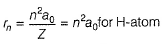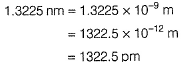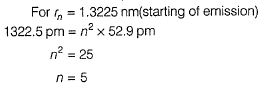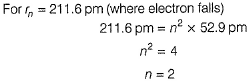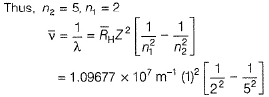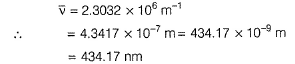QUESTION: 13

Radius of Bohr’s orbit of H-atom is 52.9 pm. An emission in H-atom starts from the orbit having radius 1.3225 nm and ends at 211.6 pm.

Q.  Spectral line appears in .......... region.

Solution:

Emission (n2 = 5 to n1 = 2) is called Balmer series and appears in visible region.

QUESTION: 14

Direction (Q. Nos. 14 and 15) Choice the correct combination of elements and column I and coloumn II are given as option (a), (b), (c) and (d), out of which ONE option is correct.

Q.

Match the equation in Column I with the name type in Column II.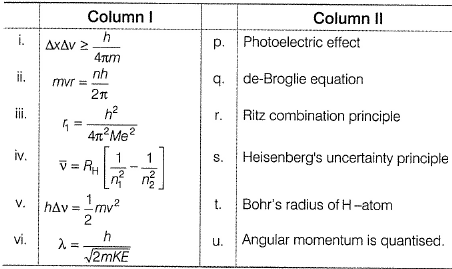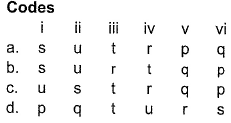Solution: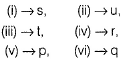QUESTION: 15

lf En = total energy, Kn = kinetic energy, Vn = potential energy and rn = radius of the nth orbit, then based on Bohr’s theory, match the parameter in Column I with the values in Column II.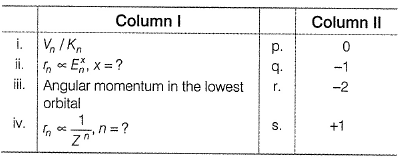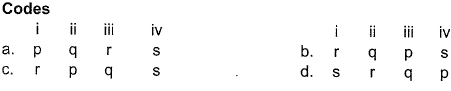Solution: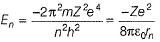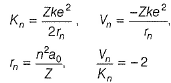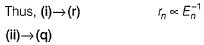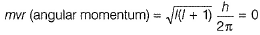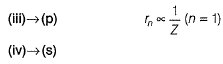*Answer can only contain numeric values
QUESTION: 16

Direction (Q. Nos. 16 - 19) This section contains 4 questions. when worked out will result in an integer from 0 to 9 (both inclusive)

Q. The energy of an electron in the first Bohr orbit of H-atom is -13.6 eV. What is the possible value of quantum number for the excited state to have energy -3.4 eV?

Solution:

E = -13.6×Z2/ n
For H atom, Z=1 and given energy = -3.4
So, we have, -3.4 = -13.6/n2
Or n = 2

*Answer can only contain numeric values
QUESTION: 17

An emission is Be3+ in observed at 2.116 A°. In which orbit is it placed?

Solution: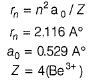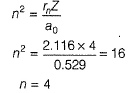*Answer can only contain numeric values
QUESTION: 18

At what minimum atomic number, a transition from n = 2 to n = 1 energy level results in the emission of X-rays with wavelength 3.0 x 10-8 m?

Solution: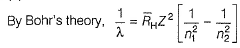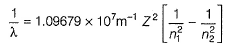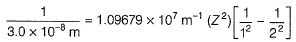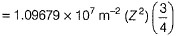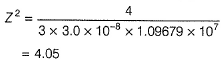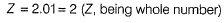*Answer can only contain numeric values
QUESTION: 19

Find the number of waves made by a Bohr’s electron in one complete revolution in its 3rd orbit

Solution: